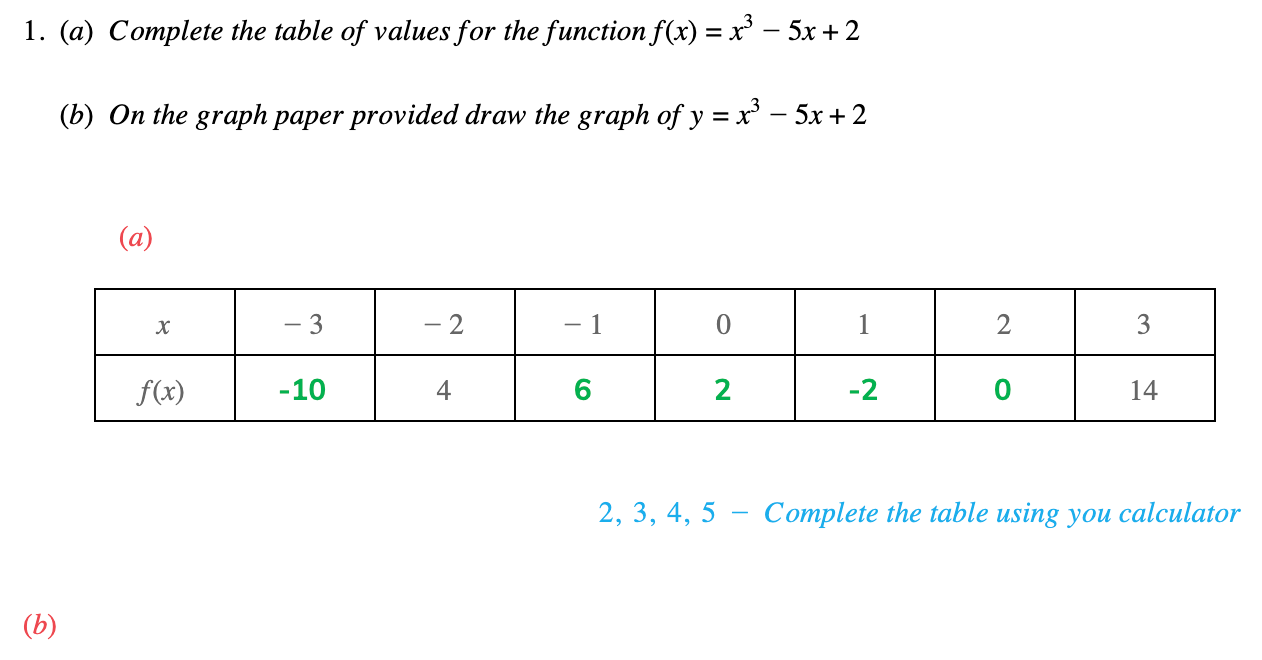# Edexcel IGCSE Maths 复习笔记 3.4.2 Drawing Graphs -Using a Table

Edexcel IGCSE Maths 复习笔记 3.4.2 Drawing Graphs -Using a Table

#### How do we draw a graph using a table of values?

• THINK what the graph might look like – see the previous notes on being familiar with shapes of graphs
• Find the TABLE function on your CALCULATOR
• Enter the FUNCTION – f(x)

(use ALPHA button and x or X, depending on make/model)(Press = when finished)

(If you are asked for another function, g(x), just press enter again)

• Enter StartEnd and Step (gap between x values)
• Press = and scroll up and down to see y values
• PLOT POINTS and join with a SMOOTH CURVE

• If your calculator does not have a TABLE function then you will have to work out each y value separately using the normal mode on your calculator
• To avoid errors always put negative numbers in brackets and use the (-) key rather than the subtraction key

#### Worked Example